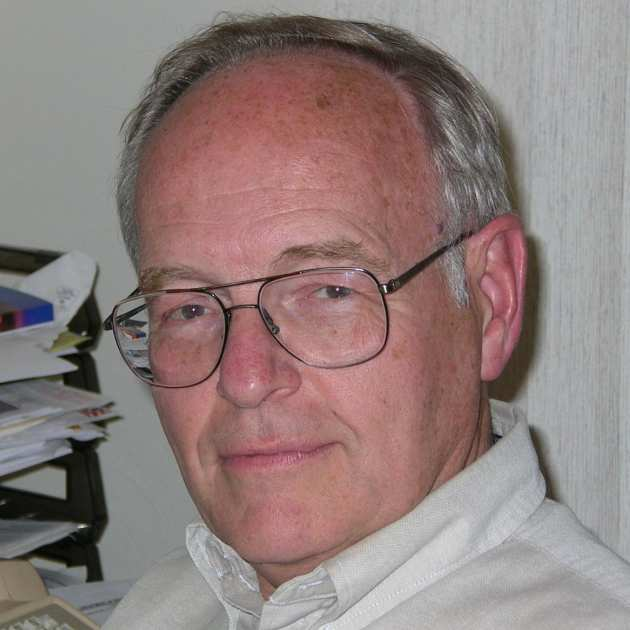###Professor Tom Kurtz will be visiting the Imperial College Mathematics Department as a Nelder. He will give a 10-hour lecture course on "Martingale problems and stochastic equations for Markov processes".

The course will be of interest to a wide range of PhD students (including the PhD students incorporated into the newly established Centres for Doctoral Training), postdocs and members of staff with research which uses stochastic equations.

## Background

Students interested in following the lectures are encouraged to review of the basics of stochastic processes and martingales in continuous time and of stochastic integration.  This background is summarized on several slides, which you can access from the following link: click here to access the slides.

Professor Kurtz will be more than happy to answer questions on any of this material.

More detail can be found in the following:

•  Chapter 2 of Ethier and Kurtz, Markov Processes: Characterization and Convergence
•  The first two chapters of Protter, Stochastic Integration and Differential Equations, Second Edition

## Abstract

Beginning with Lévy’s characterization of Brownian motion, the martingale properties of stochastic processes have proven to be fundamental in the formulation and analysis of stochastic models, in particular, in the study of Markov processes. Stochastic equations of various types provide a second fundamental approach to defining stochastic models. These lectures will explore aspects of both of these approaches and their relationship. The Martingale problem of Stroock and Varadhan will be formulated and some of its properties discussed. The notion of a weak solution of a stochastic differential equation will be introduced and the fact that solutions of appropriate Martingale problems give weak solutions of stochastic differential equations will be discussed. Time change equations for Markov chains and diffusions will be given and the equivalence of these equations to corresponding martingale problems shown.

The lectures will take place in the ICSM Building in the EPSRC Centres for Doctoral Training Suite on Level 4N. Please note that the entrance to the EPSRC CDT hub is via Sherfield building level 2 Lift lobby. There is no access to the space via the Central Library.

## Lectures:

### Lecture 1

Monday 27 April 10am-12pm Room TR3

Characterizing stochastic processes by their martingale properties:

• Lévy’s characterization of Brownian motion
• Watanabe’s characterization of the Poisson process
• Intensities for counting processes

Markov processes and their generators:

• Markov chains
• Diffusion processes
• Building Markov generators (sums of generators are generators--usually)

### Lecture 2

Tuesday 28 April 10am-12pm room TR2

Forward equations:

• Weak form
• Equivalence with martingale problems

Other types of martingale problems:

• Local martingale problems
• Constrained martingale problems

### Lecture 3

Wednesday 29 April 10am-12pm room TR3

Martingale problems for conditional distributions:

• A Markov mapping theorem
• Applications to lookdown constructions

Filtering:

• Derivation of filtering equations
• Markov property of filters
• Uniqueness

### Lecture 4

Thursday 30 April 10am-12pm room TR3

Stochastic equations for Markov processes:

• Classic models in Rd
• Equations for spatial birth and death processes

Equivalence of stochastic equations and martingale problems:

• Classic models in Rd
• Equations for spatial birth and death processes

### Lecture 5

Friday 1 May 10am-12pm room TR3

Time change equations:

• Continuous time Markov chains
• Doeblin and time-change equations for diffusions

Particle representation:

• Measure-valued processes
• Stochastic partial differential equations